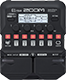# [Factory 1:5] BGN Djent

Discussion in 'Zoom G1/G1X Four' started by Tonelib, Apr 22, 2019.

1. [Factory 1:5] BGN DjentDevice: Zoom G1 Four
Firmware: 1.00

Name on device: BGN Djent
Optimized for: Phones/Speaker

Effects chain:This sharp djent sound that is suitable for drop tunings uses XtasyBlue and GoldDrive.

Effect: "ZNR" (Dynamics), active - "yes"
"Detect" = EFXIN
"Depth" = 94
"Threshold" = 100
"Decay" = 0

Effect: "GoldDrive" (Overdrive / Distortion), active - "yes"
"Gain" = 26
"Bass" = 26
"Treble" = 31
"Volume" = 62

Effect: "XtasyBlue" (Amp simulator), active - "yes"
"Bass" = 69
"Middle" = 42
"Treble" = 83
"Presence" = 72
"STRCT" = HI
"Gain" = 49
"Volume" = 53

Effect: "BGN4x12" (Cabinet), active - "yes"
"MIC" = OFF
"D57: D421" = 50
"Hi" = 71
"Lo" = 69

Effect: "Gt GEQ 7" (Filter), active - "no"
"100" = -12.0
"200" = -12.0
"400" = -4.5
"800" = -2.0
"1.6k" = 0.0
"3.2k" = 0.0
"6.4k" = 0.0
"VOL" = 80

Patch Volume: 28